# DAT Perceptual Ability : Aperture / Keyhole Questions

## Example Questions

### Example Question #1 : Aperture / Keyhole Questions

A three-dimensional object is shown in the question prompt, and outlines of five apertures or openings are shown as the answer choices. First, you imagine how the object looks from all directions (rather than form a single direction as shown). Then, pick from the five apertures outlined the opening through which the object could pass directly if the proper side were inserted first.

Here are the rules:

1. Prior to passing through the aperture, the irregular solid object may be turned in any direction. It may be started through the aperture on a side not shown.

2. Once the object is started through the aperture, it may not be twisted or turned. It must pass completely through the opening. The opening is always the exact shape of the appropriate external outline of the object.

3. Both objects are drawn to the same scale. Thus, it is possible for an opening to be the correct shape but too small for the object; however, in all cases, differences are large enough to judge by eye.

4. There are no irregularities in any hidden portion of the object; however, if the figure has symmetric indentations, the hidden portion is symmetric with the part shown.

5. For each object there is only one correct answer.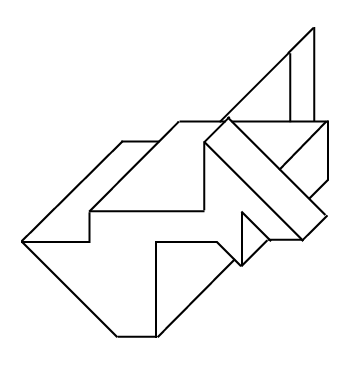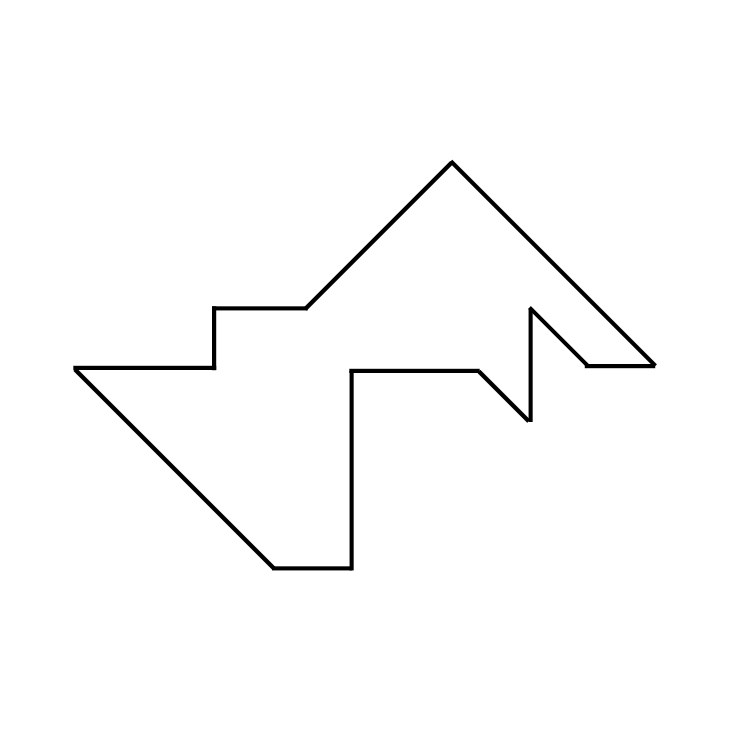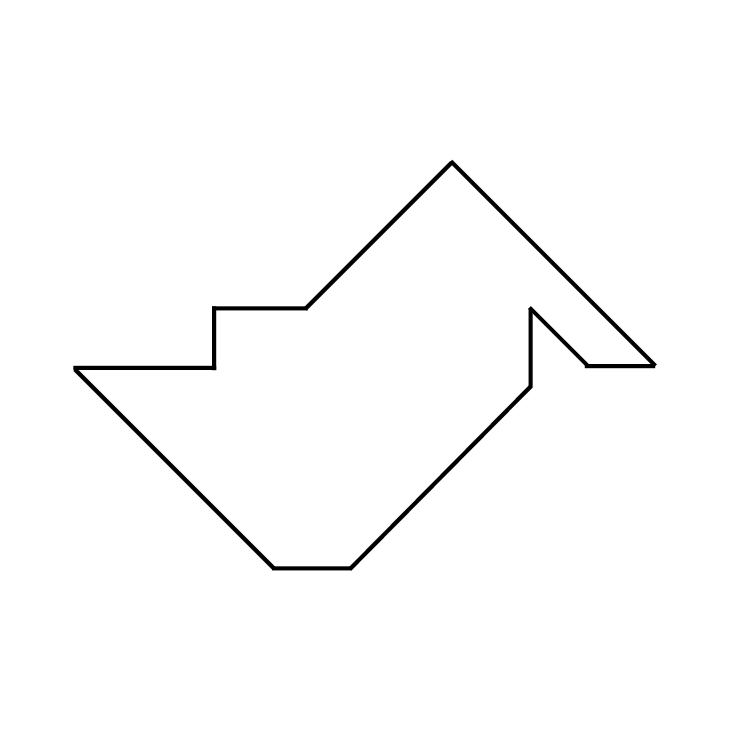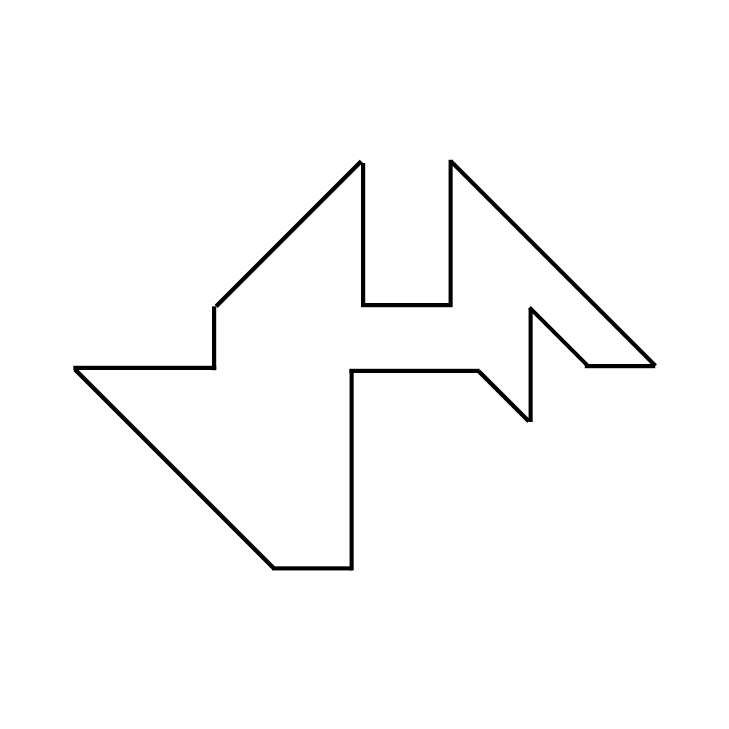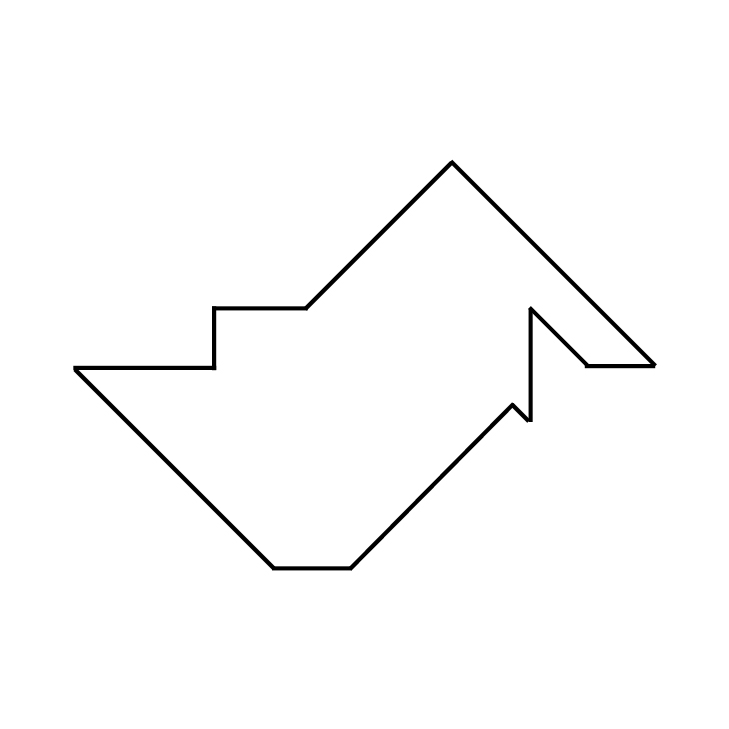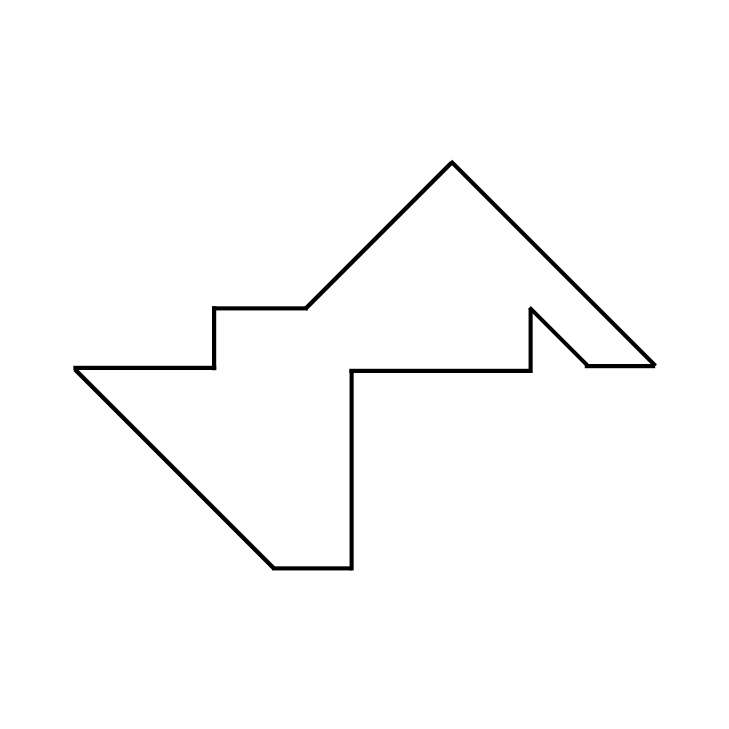Explanation:

This is the correct keyhole/aperture shape:If the three-dimensional shape were positioned so that the front-most face were parallel with the keyhole, it would be able to fit through. Note how the triangular piece that juts up above the mostly-rectangular prism farthest away from the presented three-dimensional view would require the left sloped side of the triangular portion near the top of the keyhole, and the triangular piece sticking up from the front of the presented three-dimensional view would require the right sloped side of the triangle in the keyhole. The bottom right corner of the mostly-rectangular prism comes to a point, which is accommodated by a corresponding point in the keyhole. The right angle where the mostly-rectangular prism meets the left part of the shown three-dimensional shape is also represented in the keyhole.The shape showns above are not correct because neither shows the right angle where the rectangular prism with a pointed bottom-right corner meets the left part of the shape. In addition, the shape on the right does not provide space for the pointed corner near the bottom-right of the presented view.This shape is not correct because the two triangular pieces that jut above the mostly-rectangular prism are shown with a gap in between them. By looking at the presented three-dimensional shape, you can see that they do not have this large of a gap in between their spacing on the parallel sides of the rectangular prism.This shape is not correct because it does not include space for the pointed corner of the shape similar to a rectangular prism. This is its bottom-right corner in the presented view.

### Example Question #1 : Aperture / Keyhole Questions

A three-dimensional object is shown in the question prompt, and outlines of five apertures or openings are shown as the answer choices. First, you imagine how the object looks from all directions (rather than form a single direction as shown). Then, pick from the five apertures outlined the opening through which the object could pass directly if the proper side were inserted first.

Here are the rules:

1. Prior to passing through the aperture, the irregular solid object may be turned in any direction. It may be started through the aperture on a side not shown.

2. Once the object is started through the aperture, it may not be twisted or turned. It must pass completely through the opening. The opening is always the exact shape of the appropriate external outline of the object.

3. Both objects are drawn to the same scale. Thus, it is possible for an opening to be the correct shape but too small for the object; however, in all cases, differences are large enough to judge by eye.

4. There are no irregularities in any hidden portion of the object; however, if the figure has symmetric indentations, the hidden portion is symmetric with the part shown.

5. For each object there is only one correct answer.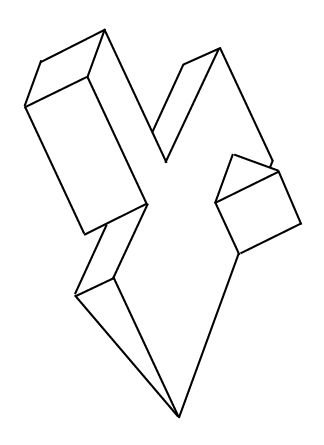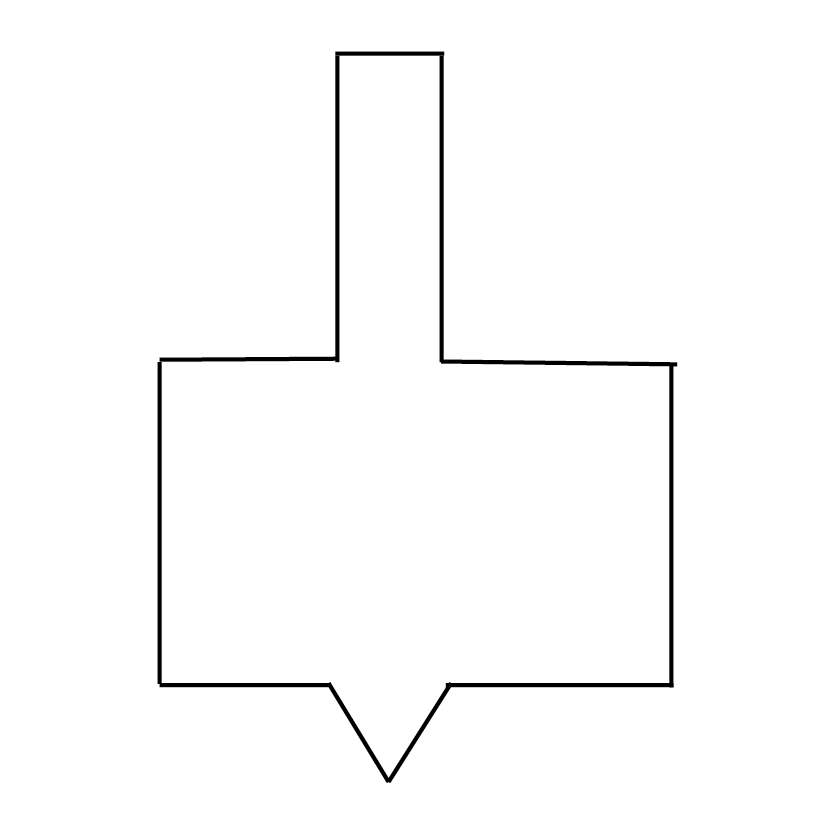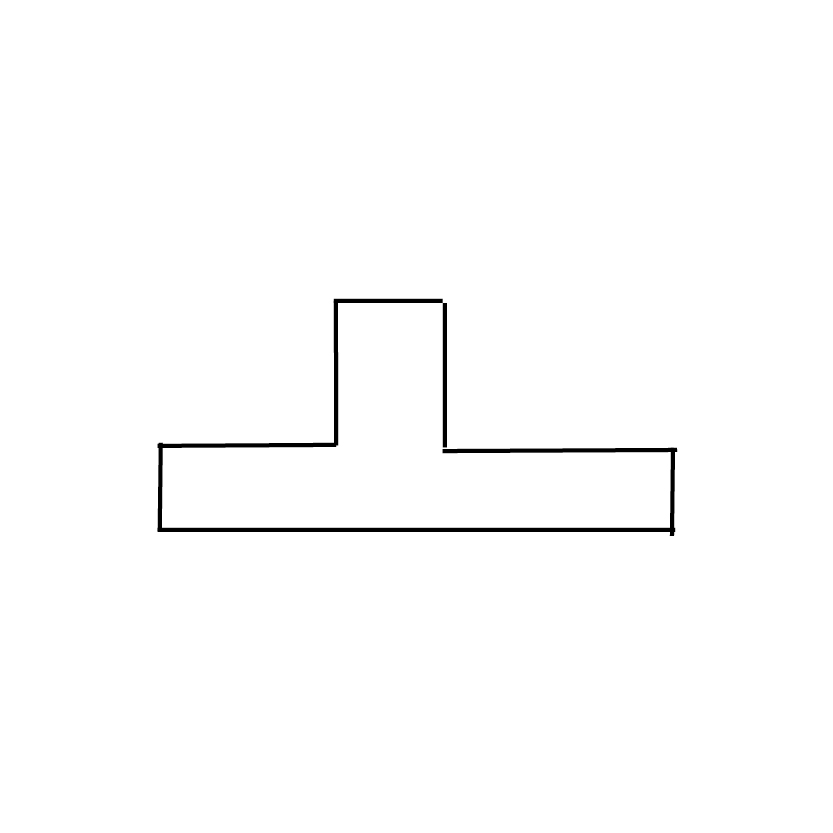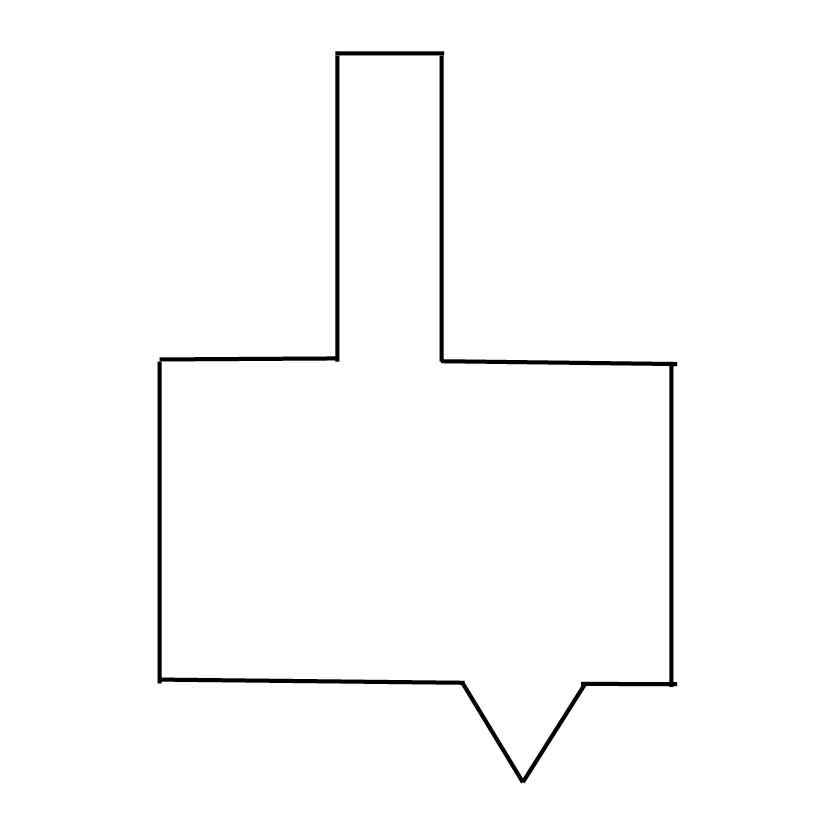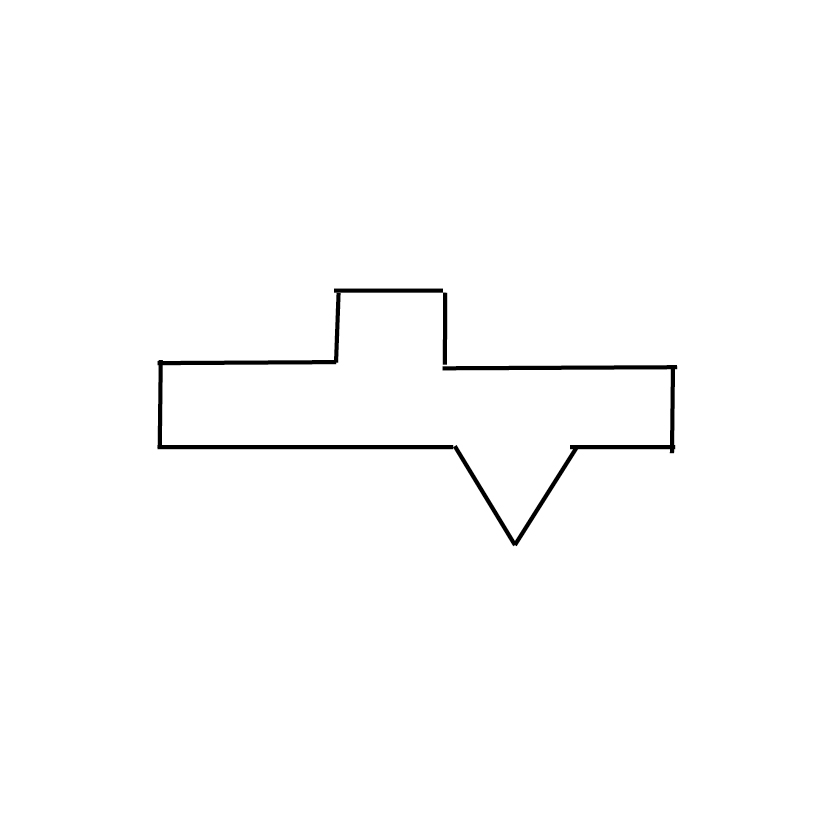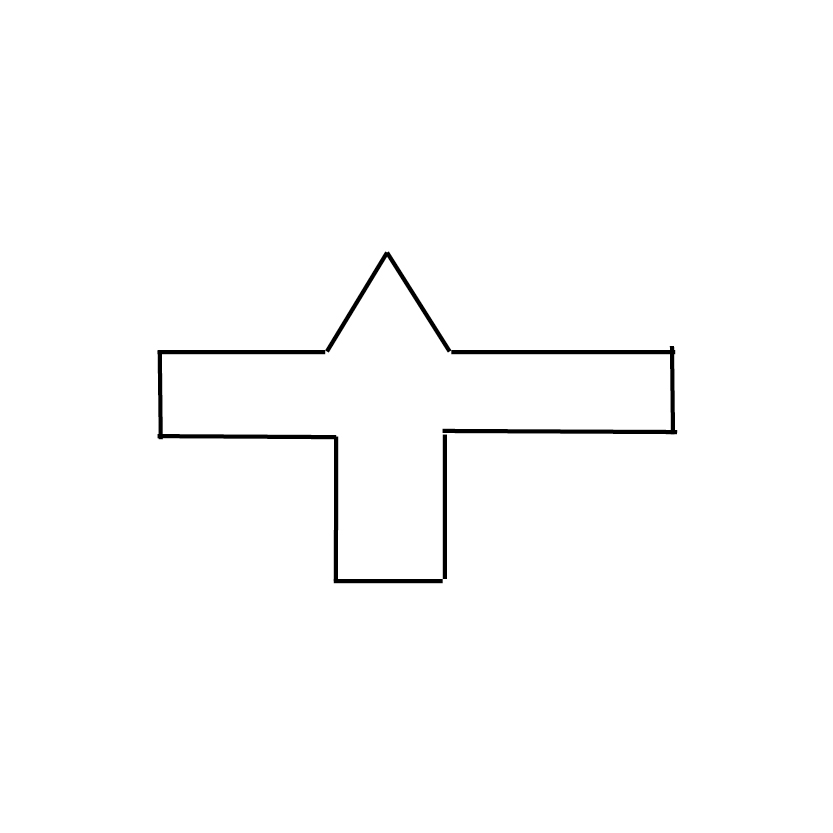Explanation:

This question deals with a shape composed of three parts. On the left of the presented three-dimensional view is a long rectangular prism; this merges on one face into a triangular prism, and on the right side of the presented view, we see that a smaller triangular prism.

The answer choices can be divided into two categories: those that involve the largest face of the large triangular prism in the middle of the shape . . .. . . and those that involve the smaller rectangular face of the triangular prism shown at the upper-left of the presented view:Consider how the smaller triangular prism relates to the largest face of the larger triangular prism. If you looked at the largest face of the larger triangular prism, would any part of the smaller triangular prism stick out of the outline? It would not, since this smaller prism is resting on that large face and doesn't stick out past its edges. We can thus ignore the two answer choices that use shapes showing the largest face of the large triangular prism, because both of these answer choices incorrectly show a triangular indent in the edge.

We now need to consider how the shape would look if we viewed it head-on from the upper-left face of the larger triangular prism. Would the rectangular prism and the smaller triangular prism both stick out from the overall rectangular outline of this face? Yes, they would. So, the middle shape shown in the three above is incorrect because it only shows an indentation for the prism and not for the triangular prism. In deciding between the remaining two shapes, remember: it doesn't matter whether the triangle or the rectangular prism is shown on the top or on the bottom of the longer rectangle. The three-dimensional shape could be rotated to make either admissible. What, then, differentiates these answers? In the shape shown on the left (or top) of the three above, the rectangular indentation is shorter than the one included in the keyhole/aperture shown on the right. In addition, the rectangular indentation and the triangular indentation are staggered, whereas in the keyhole/aperture on the far right (or bottom), they are immediately above one another.

This gives us all the information we need to answer this question correctly. You can see from the three-dimensional shape that the rectangular prism and the smaller triangular prism do not line up with one another in this way. In addition, the shorter indent for the rectangular prism makes sense, because part of its height is shared with the height of the small, upper-right face of the large triangular prism. The correct answer is the following keyhole/aperture:### All DAT Perceptual Ability Resources